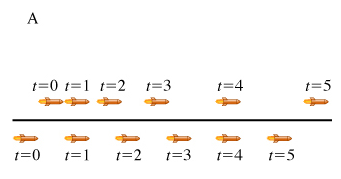# Problem: Two toy rockets are traveling in the same direction (taken to be the x axis). A diagram is shown of a time-exposure image where a stroboscope has illuminated the rockets at the uniform time intervals indicated.1. At what time(s) do the rockets have the same velocity? a. at time t=1 b. only at time t=4 c. only at times t=1 and t=4 d. at some instant in time between t=1 and t=4 e. at no time shown in the figure 2. At what time(s) do the two rockets have the same acceleration? a. at time t=1 b. only at time t=4 c. only at times t=1 and t=4 d. at some instant in time between t=1 and t=4 e. at no time shown in the figure 3. The motion of the rocket labeled A is anexample of motion with uniform (i.e., constant) __________. a. and nonzero acceleration b. velocity c. displacement d. time 4. The motion of the rocket labeled B is anexample of motion with uniform (i.e., constant) __________. a. and nonzero acceleration b. velocity c. displacement d. time 5. At what time(s) is rocket A ahead of rocket B? a. before T=1 only b. after T=4 only c. before T=1 and after T=4 d. between T=1 and T=4 e. at no time(s) shown in the figure

###### FREE Expert Solution

1.

The same velocity is achieved when the value of Δx is the same for both the rockets.

The position is same for t = 4 s

90% (166 ratings)###### Problem Details

Two toy rockets are traveling in the same direction (taken to be the x axis). A diagram is shown of a time-exposure image where a stroboscope has illuminated the rockets at the uniform time intervals indicated.1. At what time(s) do the rockets have the same velocity?

a. at time t=1

b. only at time t=4

c. only at times t=1 and t=4

d. at some instant in time between t=1 and t=4

e. at no time shown in the figure

2. At what time(s) do the two rockets have the same acceleration?

a. at time t=1

b. only at time t=4

c. only at times t=1 and t=4

d. at some instant in time between t=1 and t=4

e. at no time shown in the figure

3. The motion of the rocket labeled A is anexample of motion with uniform (i.e., constant) __________.

a. and nonzero acceleration

b. velocity

c. displacement

d. time

4. The motion of the rocket labeled B is anexample of motion with uniform (i.e., constant) __________.

a. and nonzero acceleration

b. velocity

c. displacement

d. time

5. At what time(s) is rocket A ahead of rocket B?

a. before T=1 only

b. after T=4 only

c. before T=1 and after T=4

d. between T=1 and T=4

e. at no time(s) shown in the figure